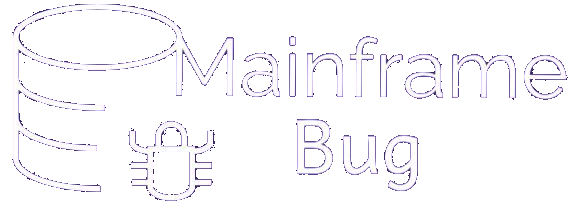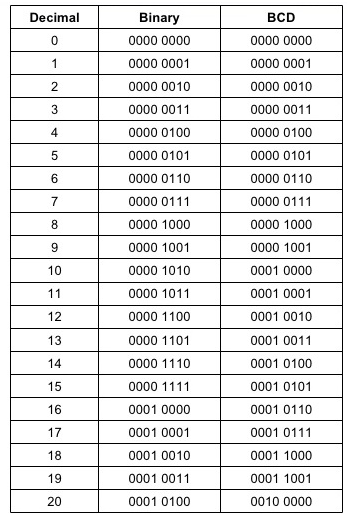## Module 5: Data Division

### Internal Data Represenataion in Mainframe

• Bit: - A bit (short for binary digit) is the smallest unit of data in a computer system. A bit has a single binary value, either 0 or 1.
• Nibble:- A nibble consists of 4 bits
• Byte:- A byte consists of 8 bits i.e. 2 nibbles
• Half word:- It consist of 16 bits i.e. 2 bytes
• Full word:- It consist of 32 bits i.e. 4 bytes
• Double word consists of 64 bits i.e. 8 bytes
• What is BCD (Binary Coded Decimal)?
• Binary coded decimal (BCD) is method of writing numerals that assigns a four-digit binary code to each digit 0 through 9 in a decimal (base-10) numeral. The four-bit BCD code for any particular single base-10 digit is its representation in binary notation, as follows:-
 0 = 0000 1 = 0001 2 = 0010 3 = 0011 4 = 0100 5 = 0101 6 = 0110 7 = 0111 8 = 1000 9 = 1001
• Numbers larger than 9, having two or more digits in the decimal system, are expressed digit by digit. For example, the BCD representation of the decimal number 1895 is :-
 0001 1000 1001 0101
• Binary VS BCD:-• Now let's see what is packed decimal and zoned decimal here